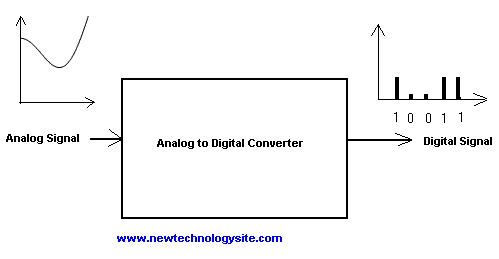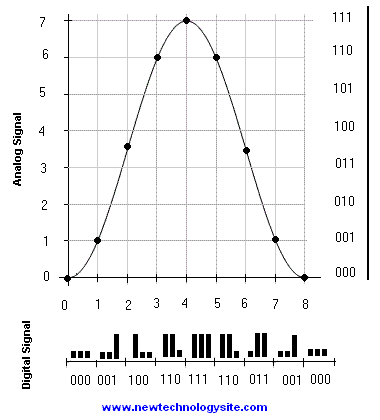# Analog to Digital Converters

How are analog signals converted into digital signals in order to be processed by computer. The principle behind converting analog signals to digital ones is explained.

## Why Analog to Digital Conversion?

Analog quantities such as pressure, sound, light change continuously and when converted to electric signal to be processed, noise generated in the electric circuits interfere with these signals. Noise has an effect on analog signals because they assume any value within a range of values. Digital signals take only two values: 1 or 0 and therefore it will be easier to differentiate between the two values even when noise interferes with these signals. So one way to protect analog signals from noise is to convert them to digital ones. There are other advantages to working with digital rather than analog signals. Digital processors are less expensive, more reliable than analog ones. Also it is relatively easier to have compatibility between digital systems than between analog systems.

It became even more important to convert analog signals to digital ones because of all the possibilities offered by the computing and processing power of computers.

The input to an analog to digital converter is an analog signal assuming continuous values within a range of value as shown in figure 1 below, and the output is a digital signal assuming two levels: 1 or 0.Fig. 1 - Analog to Digital Converter

## How to Convert Analog to Digital Signals?

In the decimal system we use 10 digits:0, 1, 2, 3, 4, 5, 5, 7, 8 and 9 to write any number (counting). It is possible to use any number of digits (3, 6 or 8) to count. The binary system uses two digits: 0 and 1 since it is easier to represent these two digits by switches having the states "ON" and "OFF". (ON for 1 and OFF for 0).

In the decimal system, 123 in expanded form is written as follows:

123 = 1 * 102 + 2 * 101 + 3 * 100

The same number in binary is expanded as follows:

123 = 1 * 26 + 1 * 25 + 1 * 24 + 1 * 23 + 0 * 22 + 1 * 21 + 1 * 20

So that 123 in decimal is written as 1111011 in binary.

Now let us suppose that we have an analog signal whose minimum level is 0 and maximum level is 7. (vertical axis figure 2 below). We now take a reading (sampling) of the signal at time t = 0, 1, 2, 3, 4, 5, 6, 7 and 8 and approximate the level of the signal to the nearest level. (horizontal axis figure 2 below). We next represent the decimal levels (0 to 7) into binary form. The table at the bottom of the page gives the time, the level of the signal at that time and the corresponding binary representation. Electronic systems are used to sample analog signal and convert it into digital form. When the binary representation is translated into a signal it becomes a digital signal in the form of pulses with level "1" and "0" that can easily be processed by computers. (see the bottom of Fig. 2)Fig. 2 - Analog Signal Converted to Digital Signal

 Time Level (rounded) Binary Representation 0 0 000 1 1 001 2 4 100 3 6 110 4 7 111 5 6 110 6 3 011 7 1 001 8 0 000